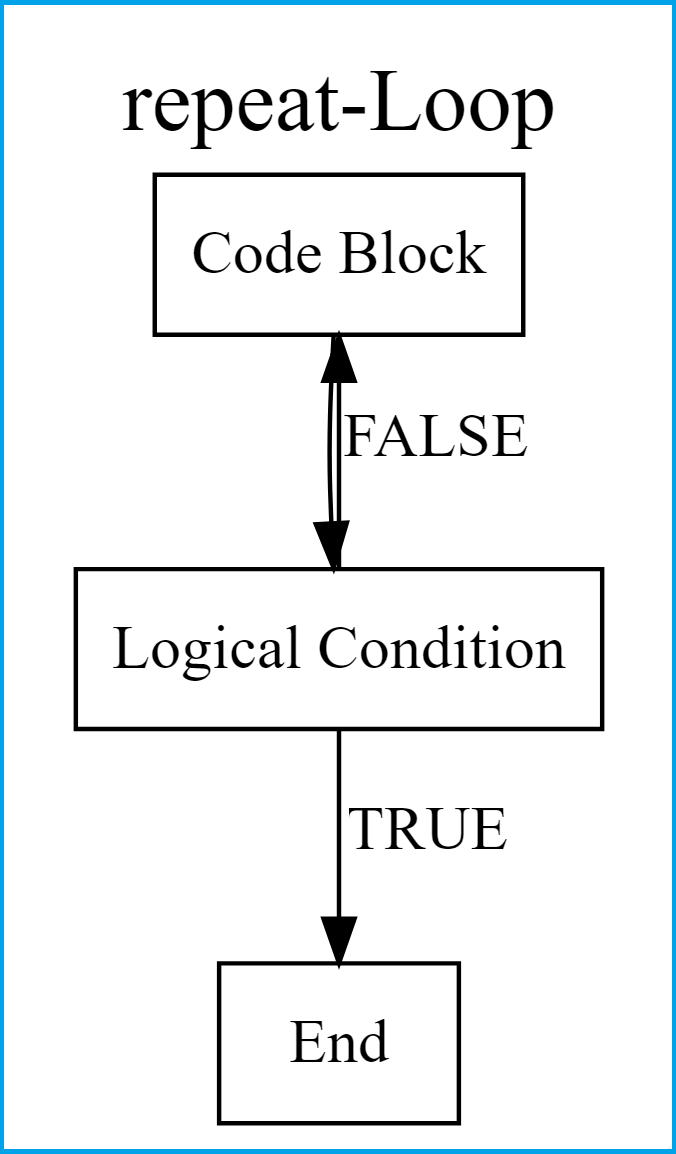# repeat-Loop in R (2 Examples) | Writing & Running repeat-Statements

In this tutorial, I’ll explain how to write and run a repeat-loop in R.

The post will consist of the following content blocks:

## Theoretical Workflow of repeat-Loops

As a first step, let’s have a look at the workflow of repeat-loops:As you can see in the previous Figure, repeat-loops start with a code block were some data manipulation or any other programming task is done. Then, it is checked whether a logical condition is met.

If this logical condition is TRUE, the break function stops the repeat-loop. If the logical condition is FALSE, the code block is run again.

## Example 1: Writing a repeat-Loop in R (Basics)

The following R code illustrates how to use and run a repeat-loop in RStudio. First, we have to create a data object that we can use in the repeat-loop later on:

```x <- 5                              # Specify example data object
x                                   # Print data object
# 5```

Our example data object is called x and contains the value 5. Now, let’s write a repeat-loop that adds plus 1 to this data object until we reach a value of 10 or larger than 10:

```repeat{                             # Starting repeat-loop

x <- x + 1                        # Main code block of repeat-loop
print(x)                          # Printing output

if(x >= 10) {                     # Logical condition of repeat-loop

break                           # Stopping repeat-loop
}
}
#  6
#  7
#  8
#  9
#  10```

Above, you can see the R syntax and the output of the repeat-loop. The repeat-loop had five iterations before the break condition was fulfilled.

## Example 2: Running repeat-Loop Over Data Frame Columns

In Example 2, I’ll explain how to run a repeat-loop through the columns of a data frame. Let’s load some example data:

```data(iris)                          # Loading exemplifying data frame
#   Sepal.Length Sepal.Width Petal.Length Petal.Width Species
# 1          5.1         3.5          1.4         0.2  setosa
# 2          4.9         3.0          1.4         0.2  setosa
# 3          4.7         3.2          1.3         0.2  setosa
# 4          4.6         3.1          1.5         0.2  setosa
# 5          5.0         3.6          1.4         0.2  setosa
# 6          5.4         3.9          1.7         0.4  setosa```

Let’s also define a running index that we can use within the repeat statement later on:

`i <- 0                              # Create running index`

Let’s go:

```repeat{                             # Start

i <- i + 1                        # Update running index

if(!is.numeric(iris[ , i])) {     # Break condition

break
}

iris[ , i] <- iris[ , i] + 100    # Change data
}```

Within the previous R code we added plus 100 to each numeric variable of our data frame until we hit the first non-numeric column. Let’s have a look at the updated data:

```head(iris)
#   Sepal.Length Sepal.Width Petal.Length Petal.Width Species
# 1        105.1       103.5        101.4       100.2  setosa
# 2        104.9       103.0        101.4       100.2  setosa
# 3        104.7       103.2        101.3       100.2  setosa
# 4        104.6       103.1        101.5       100.2  setosa
# 5        105.0       103.6        101.4       100.2  setosa
# 6        105.4       103.9        101.7       100.4  setosa```

All numeric values were increased by 100.

## Video, Further Resources & Summary

Some time ago I have released a video on my YouTube channel, which shows the R programming code of this tutorial. You can find the video below.

Furthermore, you might want to have a look at the related articles of my homepage:

Summary: In this tutorial, I showed how to use repeat-loops in the R programming language. Let me know in the comments section, in case you have any further questions.

Subscribe to the Statistics Globe Newsletter